Next: Incompressible Boundary Layers Up: Axisymmetric Incompressible Inviscid Flow Previous: Flow Around a Submerged

# Exercises

1. Demonstrate that the streamlines that pass through a stagnation point in an incompressible, irrotational, axisymmetric flow pattern cross at right angles.

2. A point source of strength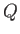is placed at the origin in a uniform stream of incompressible fluid of velocity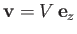. Show that the stream function of the resultant flow pattern is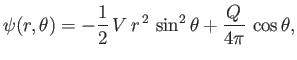(7.161)

where,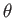,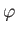are spherical coordinates. Demonstrate that the flow pattern possesses a single stagnation point at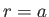,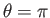, where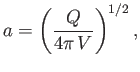and that the streamline, other than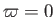, that passes through this stagnation point satisfies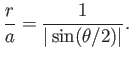The flow pattern (7.161) can be reinterpreted as that which results when a blunt obstacle lying to the right of the previously specified streamline is placed in a uniform stream of velocity. Show that the obstacle in question has the asymptotic (i.e., as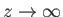) thickness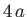. Demonstrate that the pressure distribution over the surface of the obstacle is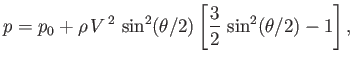whereis the fluid mass density, and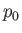the pressure at infinity. Show that the maximum pressure,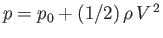, on the surface occurs at, and that the minimum pressure,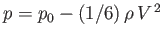, occurs at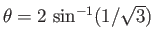. Finally, demonstrate that these are, respectively, the maximum and minimum pressures attained in the whole flow pattern.

3.and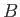are point sources of strengthsand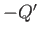, respectively, in an infinite incompressible fluid. Here,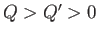. Show that the equation of a streamline is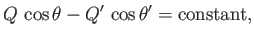where,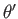are the angles that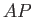,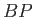make with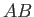.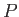being a general point. In addition, show that the cone defined by the equation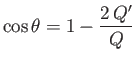divides the streamlines issuing frominto two sets, one extending to infinity, and the other terminating at. (Milne-Thompson 2011.)

4.andare point sources of equal strength(where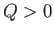) located at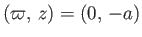and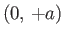, respectively in a uniform stream of incompressible fluid of velocity. Show that the stream function of the resultant flow pattern is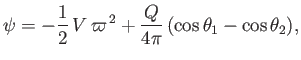(7.162)

where,are the angles that,make with.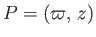being a general point. Demonstrate that the flow pattern has two stagnation points, located at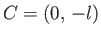and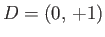, where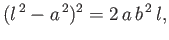and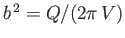. Show that the streamline (other that) that passes through these points satisfies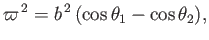and also passes through the points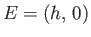and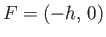, where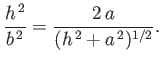The flow pattern (7.162) can be reinterpreted as that which results when an axisymmetric solid body of oval cross-section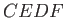, lying inside the previously specified streamline, is placed in a uniform stream of velocity. Such an obstacle is known as a Rankine solid.

5. Verify that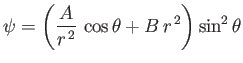is a possible stream function for an axisymmetric, incompressible, irrotational flow pattern, and find the corresponding velocity potential. (Milne-Thompson 2011.)

6. A sphere, a great depth below the surface of an incompressible fluid, is projected with velocityat an inclination ofto the horizontal. If the density of the sphere is twice that of the fluid, prove that the greatest height above the point of projection attained by the sphere is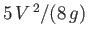. (Milne-Thompson 2011.)

7. A sphere of radius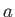is placed in an incompressible fluid flowing with the uniform velocity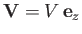. Show that the streamlines of the resultant flow pattern satisfy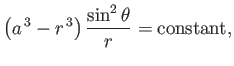where,are spherical coordinates whose origin coincides with the center of the sphere. If the sphere is divided into two halves by a diametral plane lying in the-plane, show that the resultant force between the two parts is less than it would have been if the fluid were at rest, the pressure at infinity remaining the same, by an amount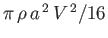, whereis the fluid density. (Milne-Thompson 2011.)

8. A sphere of radiusis moving along the-axis through an incompressible fluid with the variable speed. Show that the pressure on the surface of the sphere is least at the intersection of the surface with the plane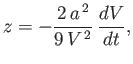the center of the sphere being instantaneously at the origin. (Milne-Thompson 2011.)

9. Consider the conformal map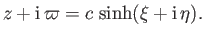where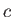is real and positive. Show that the stream function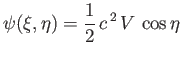can be interpreted as that of incompressible irrotational flow, with mean speed, through a circular hole of radiusin an infinite plane wall, corresponding to.Next: Incompressible Boundary Layers Up: Axisymmetric Incompressible Inviscid Flow Previous: Flow Around a Submerged
Richard Fitzpatrick 2016-03-31